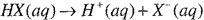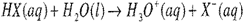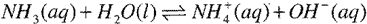Investigating Acid-Base Equilibria

Objective

To observe the pH and solubility behavior of selected chemical systems

Concepts

Acids and bases, strong and weak electrolytes, acidic and basic hydrolysis

Introduction

According to the Arrhenius model, acids increase the hydrogen ion concentration, [H+], of water, while bases increase the concentration of hydroxide ion, [OH} Thus, acids dissociate or ionize in water according to:While bases are largely restricted to metal hydroxides, which dissociate in solution according to:These definitions are only applicable to water solutions. They also make it difficult to see why a compound such as ammonia, NH3, can produce a basic solution in water. The more general model of Bronsted and Lowry identifies acids as proton donors and bases as proton acceptors, and treats all acid-base reactions as involving two reactants, one acid and one base. For our generic acid, then, the reaction that takes place is':For a strong base, one that contains hydroxide ions, the equation is essentially the same as equation (20-2), but for ammonia we have:where the ammonium ion, NH/(aq) is the conjugate acid of the weak base NH3 and hydroxide ion is the conjugate base of water, which acts as an acid by donating a proton to ammonia, leaving hydroxide ion in solution. Thus the general form of a Bronsted-Lowry acid base reaction is:

acid + base → conjugate base + conjugate acid

We sometimes observe acidic and basic behavior for substances that we wouldn't normally identify
as either acids or bases. It is those sorts of compounds that you will investigate in this experiment.

Prelaboratory Assignment

1.                 Read the entire experiment before you begin.

2.                Prepare separate data tables for Parts A-C. You can do the same for the other parts as you go.

1.                In Part C of this experiment you are directed to test the pH of several solutions by placing some of the solution in a small beaker then testing with a pH probe. Why is it not a good idea to simply insert the probe into the stock bottle?

2.                Ammonium chloride, NH4Cl, is a salt consisting of the ammonium ion, NH4+, and chloride ion, Cl-. As noted in the introduction, ammonium ion is the conjugate acid of the weak base NH3, ammonia. As such, it should react with water to produce hydronium ion and ammonia. Write the equation for this reaction.

3.                One of the reagents used in this experiment is hydroxylamine, HONH2, which can be thought of as an ammonia molecule in which one of the three hydrogen atoms has been replaced by a hydroxyl group, H-O-, with the oxygen atom bonded directly to the central nitrogen atom.

a.                 Draw the Lewis structure for hydroxylamine.

b.                The compound you will use, hydroxylammonium chloride, actually contains the conjugate
acid of hydroxylamine. Like ammonium ion, hydroxylammonium forms when a hydrogen ion
(proton) forms a coordinate covalent bond with the nitrogen atom of hydroxylamine. Draw
the Lewis structure for hydroxyl ammonium ion.

c.                 Write an equation similar to the one you wrote in Prelaboratory Question 2, showing the
reaction that occurs when hydroxylammonium ion reacts with water, producing hydronium
ion and hydroxylamine.

4.                Would the solutions produced by the reactions in Prelaboratory Questions 2 and 3 be acidic,
basic, or neutral? Explain.

5.                a.       Determine the volume of 0.50 MNa2C03 needed to precipitate all of the calcium ion from a 10.0-mL sample of 0.10 M calcium chloride, CaCl2, as calcium carbonate, CaC03.

b.                Determine the volume of 0.50 MHCI needed to convert the calcium carbonate from 5a to calcium hydrogen carbonate, Ca(HCO3)2.

Safety Precautions

1.                Chemical splash-protective eyewear must be worn at all times in the laboratory.

2.                Some of the reagents used in this experiment are toxic and/or corrosive to skin and clothing. Use care when handling them and wash your hands thoroughly with soap and water before leaving the laboratory.

Materials
Apparatus

beakers, 25-50 mL (several)
beaker, 100 mL

hot plate (or gas burner and stand)

test tubes, 150 mm or similar (several)
pH meter or interfaced pH probe

Reagents

Calcium oxide, CaO
Magnesium oxide, MgO
Phenolphthalein

Magnesium metal (ribbon or turnings)
chlorine water, Cl2(aq)

0.50 M solutions of:

Sodium acetate, NaC2H302
sodium sulfate, Na2S04

Sodium hydrogen sulfate, NaHSO4
sodium carbonate, N a2CO3

Sodium bicarbonate, NaHC03
ammonium chloride?
NH4Cl
hydroxylammonium chloride, HONH3Cl

Sodium hydroxide, NaOH(aq)
hydrochloric acid, HCl(aq)
0.10 M solutions of:

Aluminum chloride, AICl3( aq)
calcium chloride, CaCl2(aq)

Procedure

The steps that follow all involve observing the changes in pH and/or solubility that accompany various processes. For each step, your notebook record should describe what was done and what
observations you made. It is not enough to just give the step number and describe the color or pH of the system. For tests involving use of a pH meter or pH probe, it is essential that the tip of the probe be rinsed well with distilled or deionized water after each test. Failure to do so will result in collection of inappropriate data. Use a wash bottle to rinse the probe tip, catching the rinsing in a beaker.

Part A: Preliminary Tests

1.                Place a few drops of 0.50 M hydrochloric acid, HCI(aq), in each of two test tubes. Add 2-3 drops of phenolphthalein to one tube and 2-3 drops of bromothymol blue indicator to the other. Note and record the results.

2.                Repeat Step 1, but replace the 0.50 M HCl with 0.50 M NaOH. Test each with 2-3 drops of the two indicators; note and record the results.

3.                Repeat the previous steps, but this time, add 2-3 drops of each indicator to separate samples of distilled or deionized water.

Part B: Metals and Metal Oxides

4.                Place a pinch of calcium oxide in one small beaker or test tube and a pinch of magnesium oxide in another. Add about 15 mL of distilled or deionized water to each beaker and swirl to mix. Add a few drops of phenolphthalein indicator to each beaker. If no color appears, try warming each solution a bit, either with a burner or on a hot plate.

5.                Place one or two pieces of magnesium (ribbon or turnings) in a 50-mL beaker that is about half- full of distilled or deionized water. Add a few drops of phenolphthalein. If no color change is observed, try warming the mixture gently, either with a burner or on a hot plate.

Part C: Nonmetals and Nonmetal Oxides

6.                In a fume hood, put about 1 mL of chlorine water, Cl2(aq), in a small test tube and add a few drops of bromothymol blue.

7.                Place about 10 mL of ice-cold seltzer (carbonated water) in a small beaker. Add a few drops of bromothymol blue indicator.

Part D: Salts Containing Conjugate Acids of Weak Bases and Conjugate Bases of Weak Acids

8.                Using a pH meter if available, or pH test paper, try to determine the pH of 0.50 M solutions of each of the following salts: sodium acetate (NaC2H3O2), sodium sulfate (Na2SO4), sodium hydrogen sulfate (NaHSO4), sodium carbonate (Na2CO3), and sodium bicarbonate (NaHC03), also known as sodium hydrogen carbonate or baking soda. Place enough of each solution in a small beaker to cover the tip of your pH probe. If you are using pH indicator paper, about 5 mL should be enough.

9.                Again using a pH meter or pH test paper, determine the pH of 1 M solutions of ammonium chloride, NH4CI, and hydroxylammonium chloride, HONH3Cl.

10.             Repeat the process of the previous steps using a 1 M solution of ammonium acetate, NH4C2H3O2.

Part E: Effect of pH on Solubility

11.             To exactly 10.0 mL of 0.10 M CaCl2, add the volume of 0.50 MNa2CO3 that you calculated in Prelaboratory Question 5a. Stir, allowing the precipitate to settle, then determine the pH of the liquid above the precipitate.

Now add the volume of 0.50 MHCI that you calculated in Prelaboratory Question 5b. Your observations should include the results of both parts of this step. What is the pH of the mixture now?

Part F: Amphoteric Substances

12.             Place 25 mL of 0.10 M aluminum chloride, AlCl3(aq), in a 100- or 150-mL beaker. Add 5.0 mL of 0.50 M NaOH (aq) and stir. Determine the pH of the resulting mixture.

13.             Repeat the process two more times, adding 5.0-mL portions of 0.50 M NaOH, followed by stirring the mixture and determining its pH. At this point, all of the aluminum ion should have been precipitated as Al(OH)3.

14.             Finally add a fourth 5.0-mL portion of 0.50 M NaOH. Determine the pH of the system and describe its appearance.

Part A: Preliminary Tests

1.                Make a table showing the color of each of the two indicators in acidic, neutral (distilled/deionized water), and basic solution.

Part B: Metals and Metal Oxides

2.                Both magnesium metal and magnesium oxide produced pink colors, showing that hydroxide ion was produced by their respective reactions with water. Write balanced, complete ionic equations showing the reactions between magnesium and water to produce magnesium hydroxide and hydrogen gas and between magnesium oxide and water to produce magnesium hydroxide as the only product. Write a similar equation showing the reaction between calcium oxide and water.

Part C: Nonmetals and Nonmetal Oxides

3.                Molecular chlorine reacts with water to produce a mixture of hydrochloric and hypochlorous acids. Write a balanced complete ionic equation for the reaction, bearing in mind that one of the two acid products is a weak acid.       .

4.                Seltzer (carbonated water) is a solution of carbon dioxide in water. Based on the color you observed when you added bromothymol blue to seltzer, does carbon dioxide act as a Bronsted- Lowry acid or base? Write the appropriate equation for the reaction between CO2 and H2O.

Part D: Salts Containing Conjugate Acids of Weak Bases and Conjugate Bases of Weak Acids

5.                a. Identify each of the solutions from step 8 as being acidic, basic, or neutral.
b. Arrange the solutions in order of increasing pH.

6.                a. For each of the acidic solutions, the anion is the conjugate base of a weak acid. Write equations showing each of these anions acting as a Bronsted-Lowry base as it reacts with
water.

b. For each of the basic solutions, the cation is the conjugate acid of a weak base. Write equations showing each of these cations acting as a Bronsted-Lowry acid as it reacts with
water.

7.                Ammonium acetate, NH4C2H302, consists of the conjugate acid of the weak base, ammonia, and the conjugate base of the weak acid, acetic acid. Based on the observed pH of the ammonium acetate solution, what can you conclude about the relative strengths of acetic acid and ammonia?

Part E: Effect of pH on Solubility

8.          a. Write the net ionic equation for the reaction that took place when Na2CO3(aq) was added to
CaC13(aq).

b. You added just enough hydrochloric acid to the precipitate of calcium carbonate to convert all of the carbonate ion to hydrogen carbonate (bicarbonate). Write the complete ionic equation for this reaction.

Part F: Amphoteric Substances

9.                In the original aluminum chloride solution, the aluminum cation is present in the form of a hexaaquo complex, Al(H2O)63+. The first three 5.0-mL additions of NaOH converted the soluble complex to insoluble aluminum hydroxide, Al(OH)3(s). Write the complete ionic equation for the
conversion of hexaaquoaluminum to aluminum hydroxide.

10.             The final 5.0-mL portion of 0.50 M NaOH produced a new, soluble complex of aluminum and hydroxide ions.

a.                 What is the mole ratio of hydroxide to aluminum ion in this complex?

b.                Write the formula for the complex ion.